UIHistories Project: A History of the University of Illinois by Kalev Leetaru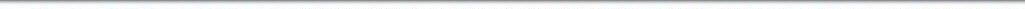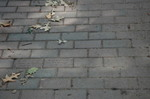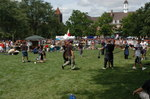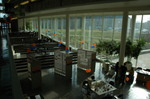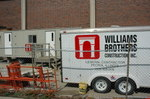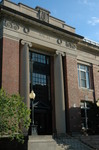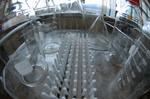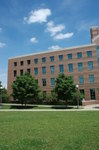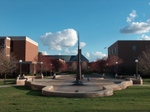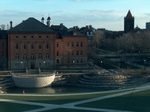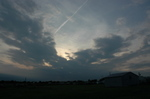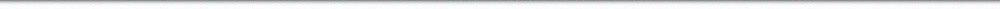N A V I G A T I O N D I G I T A L L I B R A R Y

## Repository: UIHistories Project: Board of Trustees Minutes - 1896 [PAGE 206]

Caption: Board of Trustees Minutes - 1896
This is a reduced-resolution page image for fast online browsing.

< Previous Page [Displaying Page 206 of 371] Next Page >
[VIEW ALL PAGE THUMBNAILS]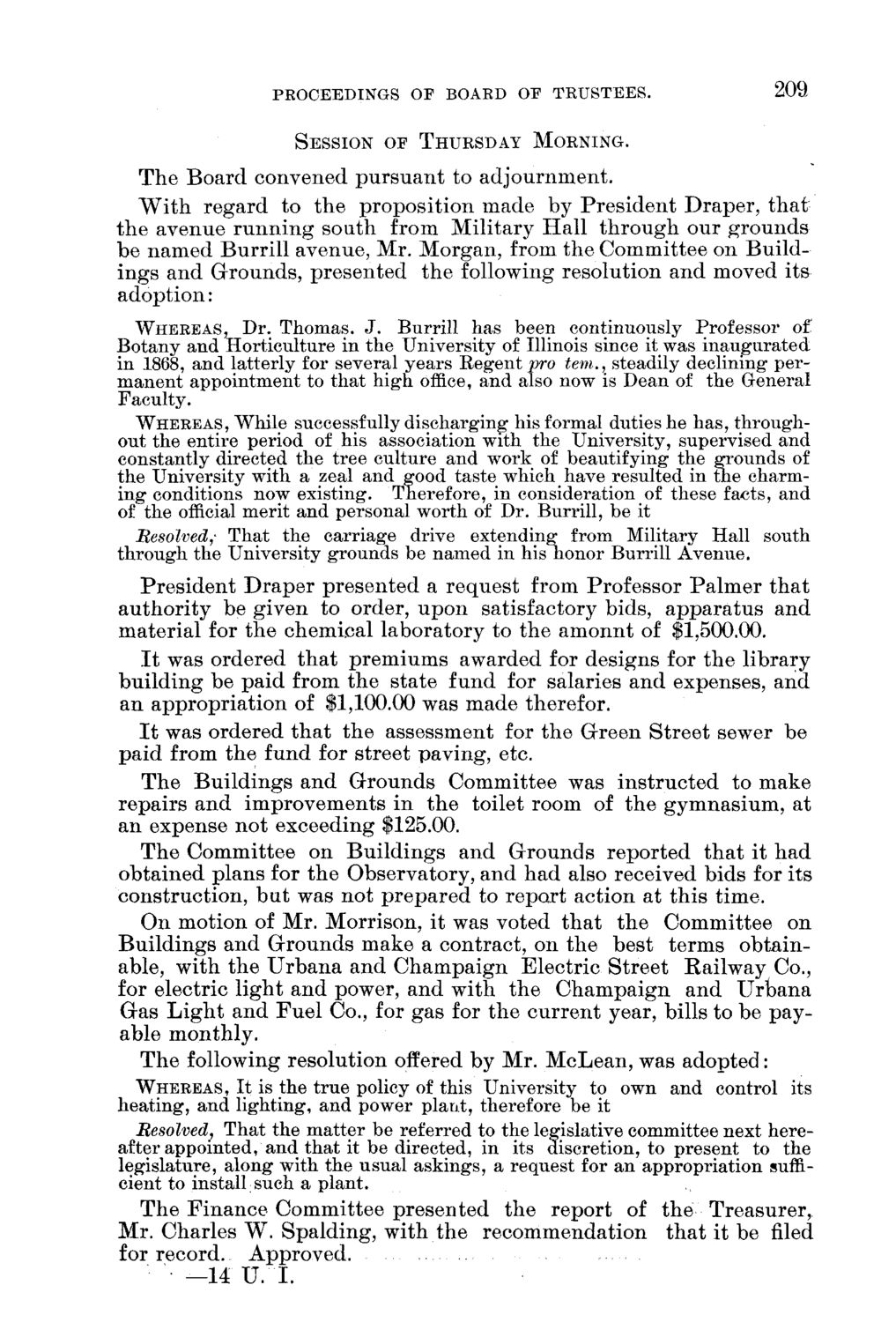### EXTRACTED TEXT FROM PAGE:

PROCEEDINGS OF BOAED OF TRUSTEES.

SESSION OF THURSDAY MORNING.

209

T h e B o a r d convened p u r s u a n t to a d j o u r n m e n t . W i t h r e g a r d to t h e p r o p o s i t i o n m a d e by P r e s i d e n t D r a p e r , t h a t t h e a v e n u e r u n n i n g s o u t h from M i l i t a r y H a l l t h r o u g h our g r o u n d s b e n a m e d B u r r i l l avenue, Mr. M o r g a n , from t h e C o m m i t t e e on B u i l d i n g s a n d G r o u n d s , p r e s e n t e d t h e following resolution a n d moved i t s adoption: WHEREAS, Dr. Thomas. J. Burrill has been continuously Professor of Botany and Horticulture in the University of Illinois since it was inaugurated in 1868, and latterly for several years Regent pro tern., steadily declining permanent appointment to that high office, and also now is Dean of the General Faculty. WHEREAS, While successfully discharging his formal duties he has, throughout the entire period of his association with the University, supervised and constantly directed the tree culture and work of beautifying the grounds of the University with a zeal and good taste which have resulted in the charming conditions now existing. Therefore, in consideration of these facts, and of the official merit and personal worth of Dr. Burrill, be it Resolved,- That the carriage drive extending from Military Hall south through the University grounds be named in his honor Burrill Avenue. P r e s i d e n t D r a p e r p r e s e n t e d a r e q u e s t from Professor P a l m e r t h a t a u t h o r i t y be given to order, u p o n satisfactory bids, a p p a r a t u s a n d m a t e r i a l for t h e c h e m i c a l l a b o r a t o r y to t h e a m o n n t of \$1,500.00. I t was ordered t h a t p r e m i u m s awarded for designs for t h e library b u i l d i n g be p a i d from t h e state fund for salaries a n d expenses, a n d a n a p p r o p r i a t i o n of \$1,100.00 was m a d e therefor. I t was ordered t h a t t h e assessment for t h e G r e e n S t r e e t sewer be p a i d from t h e fund for street paving, etc. T h e B u i l d i n g s a n d G r o u n d s C o m m i t t e e was i n s t r u c t e d to m a k e r e p a i r s a n d i m p r o v e m e n t s in t h e toilet room of t h e g y m n a s i u m , at a n expense n o t exceeding \$125.00. T h e C o m m i t t e e on B u i l d i n g s a n d G r o u n d s r e p o r t e d t h a t it h a d o b t a i n e d p l a n s for t h e Observatory, a n d h a d also received b i d s for its construction, b u t was not p r e p a r e d to r e p o r t action at t h i s time. O n motion of Mr. Morrison, it was voted t h a t t h e C o m m i t t e e on B u i l d i n g s a n d G r o u n d s m a k e a contract, on t h e best t e r m s obtainable, w i t h t h e U r b a n a a n d C h a m p a i g n E l e c t r i c S t r e e t R a i l w a y Co., for electric l i g h t a n d power, a n d with t h e C h a m p a i g n a n d U r b a n a G a s L i g h t a n d F u e l Co., for gas for t h e c u r r e n t year, bills to be p a y able m o n t h l y . T h e following resolution offered by Mr. M c L e a n , was a d o p t e d : WHEREAS, It is the true policy of this University to own and control its heating, and lighting, and power plant, therefore be it Resolved, That the matter be referred to the legislative committee next hereafter appointed, and that it be directed, in its discretion, to present to the legislature, along with the usual askings, a request for an appropriation sufficient to install such a plant. T h e F i n a n c e C o m m i t t e e p r e s e n t e d t h e r e p o r t of t h e T r e a s u r e r , M r . Charles W . S p a l d i n g , with t h e r e c o m m e n d a t i o n t h a t it be filed for record. A p p r o v e d . —14 U . I .